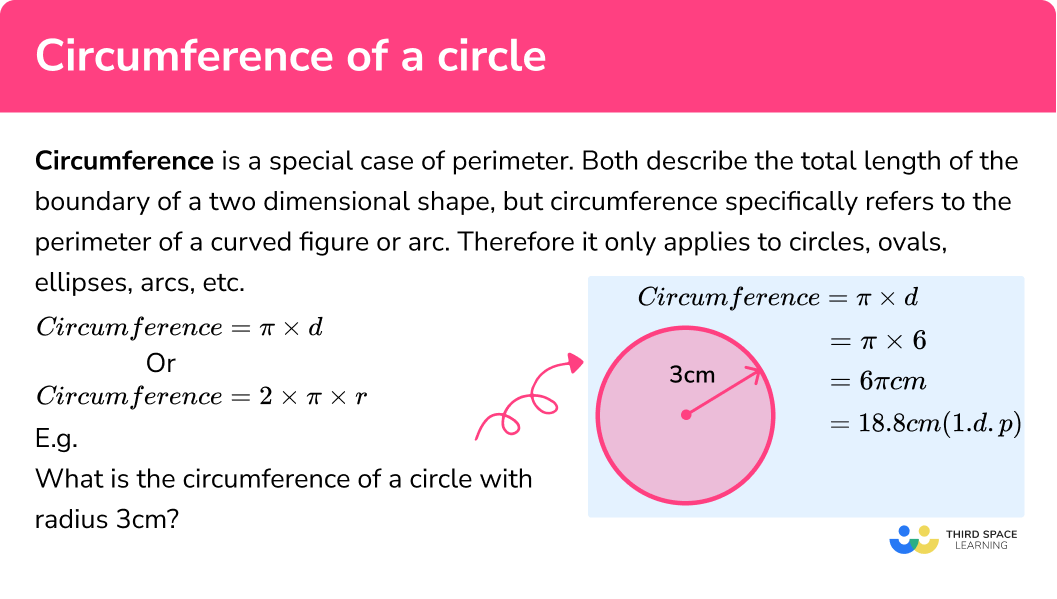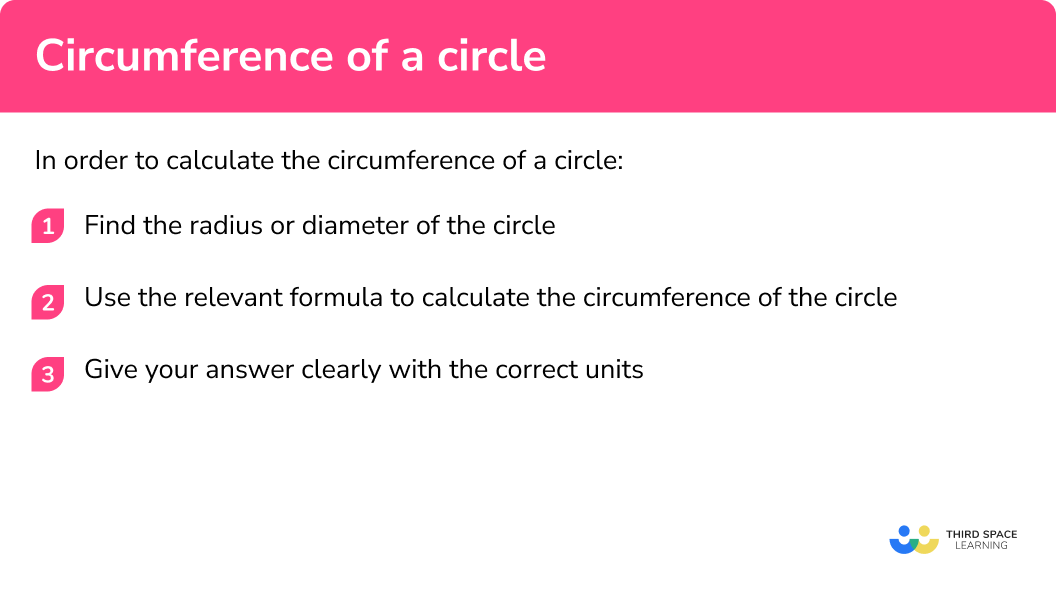GCSE Maths Geometry and Measure

Circles, Sectors and Arcs

Circumference

# Circumference Of A Circle

Here we will learn about calculating the circumference of a circle including how to calculate the circumference of a circle given the radius, diameter or area, how to calculate the perimeter of a semi-circle and how to calculate the radius or diameter of a circle given the circumference.

There are also circumference of a circle worksheets based on Edexcel, AQA and OCR exam questions, along with further guidance on where to go next if you’re still stuck.

## What is the circumference of a circle?

The circumference of a circle is the distance around the circle.

Circumference is a special case of perimeter. Both describe the total length of the boundary of a two dimensional shape, but circumference specifically refers to the perimeter of a curved figure or arc. Therefore it only applies to circles, ovals, ellipses, arcs, etc.

Circumference of a circle formula:
There is a specific relationship between the diameter of a circle and its circumference.
If you multiply the diameter of a circle by \pi you will calculate the length of the circumference. This is true of all circles.

Therefore the below formula is for the circumference of any given circle:

\pi \times d

As the diameter of the circle is twice the radius we can also use the below formula:

2\times\pi \times r

Which formula you use will depend on whether you know the diameter or the radius of the circle.

E.g.
What is the circumference of a circle with radius 3cm ?

\begin{aligned} \text { Circumference } &=2 \times \pi \times r \\\\ &=2 \times \pi \times 3 \\\\ &=6 \pi \mathrm{cm} \\\\ &=18.8 \mathrm{~cm} \quad(1 . \mathrm{d} . \mathrm{p}) \end{aligned}

OR

\begin{aligned} \text { Circumference } &=\pi \times d \\\\ &=\pi \times 6 \\\\ &=6 \pi \mathrm{cm} \\\\ &=18.8 \mathrm{~cm} \quad(1 . \mathrm{d} . \mathrm{p}) \end{aligned}

### What is the circumference of a circle?## How to calculate the circumference of a circle

In order to calculate the circumference of a circle:

1. Find the radius or diameter of the circle.
2. Use the relevant formula to calculate the circumference of the circle.

### Explain how to calculate the circumference of a circle## Circumference of a circle examples

### Example 1: calculating the circumference of the circle given the radius

A circle has a radius of 6cm .

Calculate its circumference.

1. Find the radius or diameter of the circle.

The radius is given in the question

2Use the relevant formula to calculate the circumference of the circle.

In this question you have the radius, therefore you should use the formula

\begin{aligned} &2\times\pi \times r\\\\ &2\times\pi \times 6\\\\ &12\pi \\\\ &37.699911... \end{aligned}

37.7

Remember circumference is a measure of length, therefore the units should not be “squared”

37.7cm

### Example 2: calculating the circumference of the circle given the diameter

A circle has a diameter of 10mm.

Calculate its circumference.

In this question, the question gives you the diameter.

Diameter = 10mm

\begin{aligned} &\pi \times d\\\\ &\pi \times 10\\\\ &10\pi \\\\ &31.41592... \end{aligned}

31.4

31.4mm

### Example 3: calculating the circumference of the circle, given the radius, answer in terms of 𝝅

A circle has a radius of 8m.

Calculate its circumference.

The radius is given in the question

In this question you have the radius, therefore you should use the formula

\begin{aligned} &2\times\pi \times r\\\\ &2\times\pi \times 8\\\\ &16\pi \end{aligned}

Therefore you leave the answer in the form 16 \pi

16\pi\; m

### Example 4: calculating the circumference of the circle given the diameter

A circle has a diameter of 420km.

Calculate its circumference.

In this question the question gives you the diameter.

Diameter = 420km

\begin{aligned} &\pi \times d\\\\ &\pi \times 420\\\\ &420\pi \end{aligned}

Therefore you leave the answer in the form 420 \; \pi

420\pi \; km

### Example 5: calculating the circumference of the circle given the area of a circle

A circle has a area of 21 cm^2.

Calculate its circumference.

The question gives you the area of the circle, but we need the radius/diameter in order to calculate the circumference.

We know that:

Area of a circle = \pi r^2

This means you can find the radius of the circle from the area, see below:

\begin{aligned} \text { Area }&=\pi r^{2} \\\\ \text { Area }&=21 \\\\ 21&=\pi r^{2} \hspace{2.2cm} \text{Divide both sides by } \pi \\\\ \frac{21}{\pi}&=r^{2} \hspace{2.4cm} \text{Square Root both sides of the equation} \\\\ \sqrt{\frac{21}{\pi}}&=\sqrt{r^{2}} \\\\ 2.5854 \ldots&=r \end{aligned}

Notice how we leave our answer to more decimals places than the question asks for.

This is so we do not cause a rounding error later on in the question.

In this question you now have the radius, therefore you should use the formula

\begin{aligned} &2\times\pi \times r\\\\ &2\times\pi \times 2.5854\\\\ &16.24454.. \end{aligned}

16.24

16.24cm

### Example 6: calculating the perimeter of a semi-circle given the diameter

A semicircle has a diameter of 20m.

Calculate its perimeter.

In this question the question gives you the diameter.

Diameter = 20m

\begin{aligned} &\pi \times d\\\\ &\pi \times 20\\\\ &20\pi \end{aligned}

Therefore you leave the answer in the form 20 \pi

20\pi represents the circumference of a circle with a diameter of 20m . The perimeter of the semi circle is the length of the arc and the length of the diameter added together.

Length of arc

The length of the arc is half that of the whole circle because it is a semi circle.

Therefore the length of the arc is 20\pi divided by 2.

Length of arc = 10\pi

Length of diameter

Given to you in the question, 20m

Perimeter of circle

Length of arc + Length of diameter

10\pi+20

Remember to keep it in terms of \pi as stated in the question

(10\pi+20) m

### Common misconceptions

• Not including the correct units

When working with circumference you must always give the correct units,  they should be not be squared.
E.g
cm , m, km etc.

• Not rounding correctly

These types of questions often involve rounding. You must only round at the end of the question and ensure you are rounding to what the question specifies.

•  Not giving answer in terms of \pi

Sometimes the question may ask you to give the answer ‘in terms of \pi ’. This means you do not give the numerical answer that is produced when you multiply it by \pi

E.g.

6 x \pi = 6 \pi (this is an answer in terms of pi)

6 x \pi = 18.8495592…   (this answer is not in terms of pi)

• Misuse of calculator

Ensure you know how to correctly use the \pi button on your calculator

Circumference of a circle is part of our series of lessons to support revision on circles, sectors and arcs. You may find it helpful to start with the main circles, sectors and arcs lesson for a summary of what to expect, or use the step by step guides below for further detail on individual topics. Other lessons in this series include:

### Practice circumference of a circle questions

1. A circle has a diameter of 6cm . What is the circumference of the circle in terms of \pi ?

18.8cm6\pi cm^218.8m6\pi cm\begin{aligned} &\pi \times d \\ &= \pi \times 6 \\ &=6\pi cm \end{aligned}

2. A circle has a diameter of 6cm . What is the circumference of the circle to 1 decimal place?

18.8cm6\pi cm^218.8m6\pi cm\begin{aligned} &\pi \times d \\ &= \pi \times 6 \\ &= 18.849… cm \end{aligned}

18.8 cm (rounded to one decimal place)

3. Which of these could be the circumference of a circle?

31.4 \pi cm^220m^210\pi cm24.5 cm^3Circumference is a measure of length and this is the only answer with a measure of length (cm) as its units.

4. A circle has a radius of 1cm. What is its circumference to 1 decimal place?

\pi cm^2\pi cm6.3cm6.28cm\begin{aligned} &2 \times \pi \times r \\ &= 2 \times \pi \times 1 \\ &= 6.2831… cm \end{aligned}

= 6.3 cm (rounded to one decimal place)

5. A circle has a radius of 1cm . What is its circumference in terms of \pi ?

2\pi cm^22\pi cm6.3cm6.28cm\begin{aligned} &2 \times \pi \times r \\ &= 2 \times \pi \times 1 \\ &= 2\pi cm \end{aligned}

This answer has been left in terms of \pi

6. A circle has a diameter of 4cm . What is its circumference in terms of \pi ?

4\pi cm\pi cm12.7cm12.7 cm^2\begin{aligned} & \pi \times d \\ &= \pi \times 4 \\ &= 4\pi cm \end{aligned}

7. A circle has a radius of 100cm . What is its circumference to the nearest whole number?

628.3 cm^2629 cm628 cm200\pi cm\begin{aligned} & 2 \times \pi \times r \\ &= 2 \times\pi \times 100 \\ &= 628.3185.. cm \end{aligned}

=628 cm   (rounded to the nearest integer)

Remember integer means whole number

### Circumference of a circle GCSE questions

1. The radius of a circle is 3.5 cm , work out the circumference of the circle.

(3 marks)

2 \times \pi \times3.5   or  21.9911… seen

(1)

22.0

(1)

cm

(1)

2. The radius of a circle is 17.2 m , calculate the circumference of the circle.

(3 marks)

2 \times \pi \times17.2   or  108.0707… seen

(1)

108.07

(1)

cm

(1)

3. The diameter of a circle is 12mm , work out the circumference of the circle.

(2 marks)

\pi \times 12

(1)

12\pi cm

(1)

4. The diameter of a circle is 34cm , work out the circumference of the circle.

(2 marks)

\pi \times 34

(1)

34\pi cm

(1)

5. The area of a circle is 72cm , work out the circumference of the circle.

(4 marks)

r = \sqrt{\frac{72}{\pi}}   or    4.787… seen

(1)

2 \times \pi \times 4.787

(1)

30.0776…

(1)

30 cm

(1)

6. A tile is in the shape of a semicircle has a perimeter of 15cm .
Work out the length of the diameter. Give your answer correct to one decimal place.

(5 marks)

Seen or implied that the perimeter of a semicircle is the diameter added to the length of the arc. Could be seen in a diagram.

E.g.

Diameter + half of the circumference = 15

(1)

Seen or implied that the length of the arc half of the total circumference

E.g.

\frac{\pi d}{2} seen

(1)

Correct method to find the diameter of the circle e.g.

d = \frac{15}{1+\frac{\pi}{2}}

(1)

5.834…

(1)

5.8 cm

(1)

7. The circumference of a circle is 64\pi cm .
Calculate the radius of the circle.

(2 marks)

64\pi = 2\times\pi \times r

(1)

32 = r

(1)

## Learning checklist

You have now learned how to:

• Identify and apply circle definitions and properties, including: centre, radius, chord, diameter, circumference
• Know the formulae for circumference of a circle
• Give answers in terms of \pi
• Calculate circumference of circles and the perimeter semi-circles

## Still stuck?

Prepare your KS4 students for maths GCSEs success with Third Space Learning. Weekly online one to one GCSE maths revision lessons delivered by expert maths tutors.

Find out more about our GCSE maths tuition programme.